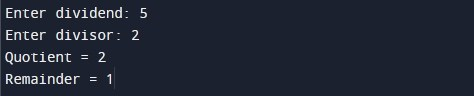C Program to Find Quotient and Remainder

In this tutorial you will learn about the C Program to Find Quotient and Remainder and its application with practical example.

In this tutorial, we will learn to create a program to find the quotient and remainder when an integer number is divided by another integer number using C programming.

Prerequisites

Before starting with this tutorial we assume that you are best aware of the following C programming topics:

• C Data Types
• C Variables
• C Input Output
• C Operators

Program to Find Quotient and Remainder

In this program we will find quotient and remainder when an integer number is divided by another integer number. In this program user is first asked to enter two integers (dividend and divisor). Next, we will compute the quotient and remainder based on the input numbers. Later in the program we will display quotient and remainder.

Output:-In the above program, we have first declared and initialized a set variables required in the program.

• num1 = it holds the dividend value
• num2 = it holds the divisor value
• quotient = it holds the quotient
• remainder = it holds the remainder

In the next statement user will be prompted to enter the two integer number values which will be assigned to variable ‘num1’ and ‘num2’ respectively. Next,we will computes quotient using division operator ( / ) and remainder using modulus operator ( % ). Finally, we will be displaying the quotient and remainder of the provided numbers using printf statement with corresponding format specifier.

Program to find Quotient and Remainder using function

In this program we will find quotient and remainder of given numbers using function. We would first declared and initialized the required variables. Next, we would prompt user to input two integers (divisor and dividend) and the quotient and the remainder computed using user defined function and later we will display quotient and the remainder.

Output:-In the above program, we have first defined two function as following:

findQuotient() :- Function to computer quotient

findRemainder() :- Function to computer remainder

Next, we have declared and initialized a set variables required in the program.

• num1 = it holds the dividend value
• num2 = it holds the divisor value
• quotient = it holds the quotient
• remainder = it holds the remainder

In the next statement user will be prompted to enter the two integer number values which will be assigned to variable ‘num1’ and ‘num2’ respectively. Next,we will calling findQuotient() and findRemainder() to compute and return quotient and remainder respectively. We have assigned resultant quotient and remainder to ‘quotient’ and ‘remainder’ variables respectively.Finally, we will be displaying the quotient and remainder of the provided numbers using printf statement with corresponding format specifier.

In this tutorial we have learn about the C Program to Find Quotient and Remainder and its application with practical example. I hope you will like this tutorial.